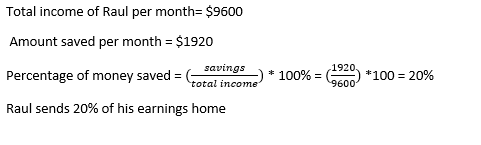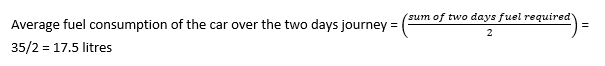# Tech Mahindra Quantitative Ability Previous Year Questions

09 January 2023

Tech Mahindra Quantitative Ability Previous Year Questions

Tech Mahindra Quantitative Ability Questions

Q1) On buying an article for Rs. 300 and then selling it, a shopkeeper incurs a loss of 11%. If the customer sold the same article to his friend for a profit of 10%, what was the price at which the customer sold the article to his friend?

A. Rs. 293.70

B. Rs. 299.9

C. Rs. 240.5

D. Rs. 297.50

Explanation:

Cost price of the article= Rs. 300

Selling price of the article to the customer=(100-11) % of cost price of article.

= (89/100) ×300=267.

Now the cost price of the article for the customer is Rs. 267

Customer makes a profit of 10%.

Selling price of the article by the customer to his friend is (110/100) ×267=293.7

Therefore, the customer sells the article at Rs. 293.7 to his friend.

Q2) Raul earns \$9,600 per month. He saves \$1,920 per month and sends it back home. What percentage of his total earnings does he send back home every month?

A.17.5%

B.20 %

C.25 %

D.15 %

Explanation:Q3) Working together Andy and Angela can complete a work in 6 days. Working individually Andy can complete the same work in 10 days. What time can Angela take to complete the work alone?

A.12

B.15

C.13

D.14

E.16

Explanation:Q4) A boy has denominations of ten cents, twenty cents and one dollar coins in the ratio 10:17:7 respectively in his piggy bank. On counting it was found that the total amount was \$57. How many coins of denomination 20 cents were there in the piggy bank?

A.114

B.100

C.85

D.95

Explanation: Let's say, no. of coins of 10 cents = x

No. of coins of 20 cents = 1.7x

No. of coins of one dollar = 0.7x

One dollar is equal to 100 cents i.e., 10 cents = 0.1 dollar

0.1*x+0.2*1.7x+1*0.7x = \$57

1.14*x = 57

X = 50

No. of coins of 20 cents = 1.7*x

=1.7*50

=85

Q5) The average of five quantities is 6. The average of two of them is 3. What is the average of the remaining three quantities?

A.6.5

B.8

C.6

D.8.5

Explanation: Average of five quantities = 6.

Then, sum of five quantities = 30.

Average of two of them is 3, then sum of two of them is 6.

From the above statement, sum of the remaining three quantities = 24.

Then, average of the remaining three quantities = 24/3 = 8.

Q6) Raja buys some apples at the rate of four for a rupee and same numbers of oranges at three for a rupee. To make a profit of 25%, Raja should sell 6 apples for .

A. 5.75

B. 6.75

C. 3.75

D. 4.75

Explanation: let Raja buys ‘x’ apples at rate four for a rupee and ‘x’ apples at three for a rupee.

So, cost price = x/4 + x/3

Then, CP = 6/4 + 6/3 = 3.5

Now SP = (125/100) *3.5 = 4.75

Therefore, Raja should sell 6 apples for 4.75

Q7) Prasanth bought an article at a 25 percent discount on the labeled price. He again sells the article at 20 percent on labeled price. Find the percent profit earned by Prasanth in the whole transaction

A. 50%

B. 60%

C. 30%

D. 40%

Explanation: Let the labelled price is 100.

Prasanth bought it for 75 after getting a 25% discount.

Now he sells the article at (120/100) *100 = 120.

So % profit he earns = (45/75) *100 = 60%

Q8) Due to my good performance at work, I received three successive increments of equal percentage. My salary increased from Rs.100 to Rs.133.1. By what percentage is my salary raised each time?

A. 12%

B. 10%

C. 28%

D. 19%

Explanation: This question can be solved through option verification,

First option – 12%

After first increment, 100 + 12%100 = 112

Then second increment, 112 + 12%112 = 125.44

Then last which is third increment, 125.44 + 12%125.44 = 140.49, which is not satisfying the condition of increasing to 133.1 after three successive increments.

Similarly, Second option – 10%

After first increment, 100 + 10%100 = 110

Then second increment, 110 + 10%110 = 121

Then last which is third increment, 121 + 10%121 = 133.1, which satisfies the given condition. So, option B is the answer.

Q9) If a car requires 15 liters of fuel on day 1 of the journey and 20 liters of fuel on day 2, then what is the average fuel consumption of the car over the two days journey?

A. 17.5 liters

B. 18 liters

C. 19 liters

D. 20 liters

Explanation:Q10) Twenty men can complete a piece of work in 25 days. In how many days can 5 men complete the same work?

A. 50

B. 40

C. 100

D. 150

Explanation: W = m × d

m1 × d1 = m2 × d2

Where -

m1 = Initial men

d1 = Initial days

m2 = Final men

d2 = Final days

Thus,

25 × 20 = 5 × d2

d2 = 25 × 20/5

d2 = 5 × 20

= 100

Q11) An aircraft flying at the height of 240 m above sea level drops a bomb on a submarine lying 420 m below sea level. The bomb falls down at the average speed of 60 m per second. How much time will it take to strike the submarine?

A. 3 seconds

B. 6 seconds

C. 11 seconds

D. 12 seconds

Explanation: Height above sea level at which the aircraft flies = 240 m

Depth below sea level at which the submarine is lying = 420m

Average speed with which the bomb falls down = 60 m/s

Total distance the bomb falls to strike the submarine = 240 +420 = 660m

As we know,

Time = Distance/speed = 660/60 = 11 seconds.

Q12) I have bought a second-hand car for Rs. 2,50,000. I have to pay 2% of the purchase price towards the annual maintenance cost and an annual tax of Rs. 2,000. At what monthly rent should I lend the car to a taxi driver to get a 15% return on net investment of one year?

A. Rs. 3,350.50

B. Rs. 3,212.50

C. Rs. 3,412.50

D. Rs. 3,550.50

Explanation: The total cost for the year = 250000 + 2% of 2500000 + 2000
= Rs. 257000
For getting return of 15% he must earn = (257000×15)/100

= Rs. 38550 per year
Then, Monthly Rent = 38550/12

= Rs. 3212.5

Q13) What is the ratio of the father’s age to the son’s age, if their ages are 35 years and 5 years respectively?

A. 5 : 1

B. 6 : 1

C. 7 : 1

D. 1 : 7

Explanation: Given Father’s age = 35 years

And Son’s age = 5 years

Then, ratio of father’s age to the son’s age = 35 : 5 = 7 : 1

Q14) Three years ago, the average age of a family of five members was 17 years. After a baby is born, the average age of the family is the same today. What is the age of the baby?

A. 1 year

B. 2 years

C. 6 months

D. 9 months

Explanation: Total age of the family three years ago = 17 x 5 = 85 years.
Let the present age of the child be x years.
Present total age of the family = 85 + 5 x 3 + x = (100 + x) years.
Given (100+x)/? 66 =,17
100+x=102

x=2 years

Q15) A sum of money was invested for 3 years at the rate of 4% per annum compounded annually amounted to \$84,364.80. What is the sum invested?

A. \$65,000

B. \$70,000

C. \$75,000

D. \$80,000

Explanation: A = P (1 + R/100) n

A = \$84,364.80

P = ?

R = 4

n = 3

84,364.80 = P (1 + 4/100) ³

84,364.80 = P (1.04) ³

84,364.80 = P (1.124864)

P = \$75,000

###